Aegidius
Plüssin examples
Use the asterisk (*) character for wildcard searches
Searches on more than one word will be treated "as a phrase"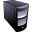Server Status
www.aplu.ch
www.java-online.eu
Print TextJava exemplarisch (Textbook, in German)Programming Concepts in Python Tutorial and Exercises in German, French and EnglishTCPCom An event-driven socket libarry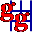JGameGrid        (Java Game Framework)JCardGame (JGameGrid Card Game Addon) Ported to AndroidJDroidLib     NEW: Support for Smartphone IDE (JGameGrid/TcpJLib for Android)JTurtleLib     (Java Turtle Graphics for Android)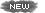Python-Exemplary Raspberry Pi Tutorial (in English and German)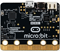New micro:bit     Single-board Computer Python Tutorial (Now released!)BrickPiLib Library for the BrickPi KitRaspiBrick Low-Budget Robotics with the Raspberry Pi EV3JLib (Library for Lego EV3 Robot) Support for Jython & AndroidTcpJLib (TCP/IP Communication)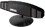KinectJLib (Kinect Controller Library for Java)JAW       (Java API Wrapper, 32/64bit)Python/Jython (Using TigerJython IDE)Java Utilities  (Graphics, Turtle)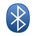Bluetooth Lib (For J2ME/J2SE)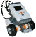NxtJLib (Library for Lego NXT Robot) Support for J2SE/J2ME & AndroidXboxController (Xbox Gamepad Library)Simulations    (for Physics Teachers)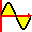Overtone           (Spectral Composition/Analysis)FtController  (Fischertechnik Java Library) CoverSheet      (Presentation Tool) Gidlet      (Java ME Framework) MidPak      (MIDlet Packaging) JavaOC      (Java Object Creator) JawGadget (Transparent Windows) C++/Champ      (Graphics Framework) Contact Home
JythonThe class GPanel provides a graphics window with a user definable floating point coordinate system. Elementary methods are provided to draw shapes like lines, circles, rectangles, etc. It is used for simple animations and simulations, especially in the field of testing graphics algorithms, simulation of physical systems and education.

The ch.aplu.util package (JavaDoc) contains several useful helper classes, among them GPanel, and is integrated in the current release of TigerJython. The import statement inserts all methods of GPanel into the global namespace (JavaDoc).

In the first example we draw a famous Moiré pattern with just a few lines of code.

```# GPanelEx1.py

from gpanel import *

makeGPanel(0, 10, 0, 10)

for i in range(0, 11):
for k in range (0, 11):
line(i, 0, k, 10)
delay(100)

for i in range(0, 11):
for k in range (0, 11):
line(0, i, 10, k)
delay(100)
```Select GPanelEx1.py (Ctrl+C to copy, Ctrl+V to paste)
Execute the program locally using WebStart.

Discussion: makeGPanel(() takes four float parameters xmin, xmax, ymin, ymax that define the user coordinate system (origin and range). As usual in mathematics the x-axis is positive to the right and the y-axis positive to the top. For many graphics applications, especially in the field of simulations, a floating point user coordinate system simplifies and cleans up the code, but you may get one pixel rounding errors.

Because we want to see how the program works, we slow down the program by calling delay(100) after drawing a single line. You may remove the delay and enjoy the full speed.

In the next example the computer is a painter that draws randomly colored polygons with a random number of vertices at random positions.

```# GPanelEx2.py

from gpanel import *
from ch.aplu.util import X11Color
from random import choice
import random

NB_POLYGONS = 20
makeGPanel()
for i in range(NB_POLYGONS):
color = getRandomX11Color()
setColor(color)
m = random.randrange(5, 10)
x = [0.0] * m
y = [0.0] * m
for k in range(m):
x[k] = random.random()
y[k] = random.random()
fillPolygon(x, y);
delay(100)
```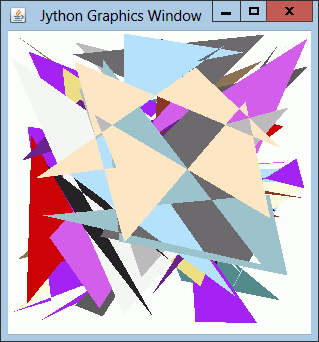Select GPanelEx2.py (Ctrl+C to copy, Ctrl+V to paste)
Execute the program locally using WebStart.

Discussion: We select a random color from the X11 Color palette using the getRandomX11Color() function. The number m of the polygon vertices is randomly chosen in the range between 5 and 10. The lists x and y of size m are created and filled with random numbers between 0 and 1. The lists are then passed to the fillPolygon() method that interprets the list entries as coordinates of the polygon vertices. Again we slow down the painting by calling delay(100).

The next example shows the conformal mapping of the complex plane using the inversion z -> 1/z. An equidistant grid in the range (-5, 5) for the real and imaginary axis is transformed. Because Python has a built-in complex number type and we use a coordinate system adapted to the problem, the program is of comparable complexity as when using a specialized math and graphics software like Mathematica.

```# GPanelEx3.py

from gpanel import *
import math

def transform():
# Transform horizontal grid lines
setColor("green")
z = complex(min, min)
while z.imag < max:
z = complex(min, z.imag)
cmove(f(z))
while z.real < max:
cdraw(f(z))
z = z + reStep
z = z + imStep
repaint()

# Transform vertical grid lines
setColor("red")
z = complex(min, min)
while z.real < max:
z = complex(z.real, min)
cmove(f(z))
while z.imag < max:
cdraw(f(z))
z = z + imStep
z = z + reStep
repaint()

# function f(z) = 1/z
def f(z):
if abs(z) != 0:
return 1 / z
else:
return complex(0, 0)

def cmove(z):
move(z.real, z.imag)

def cdraw(z):
draw(z.real, z.imag)

min = -5.0
max = 5.0
step = (max - min) / 400.0
reStep = complex(step, 0)
imStep = complex(0, step)

makeGPanel(min, max, min, max)
line(min, 0, max, 0)  # Real axis
line(0, min, 0, max) # Imaginary axis
enableRepaint(False)
transform()
```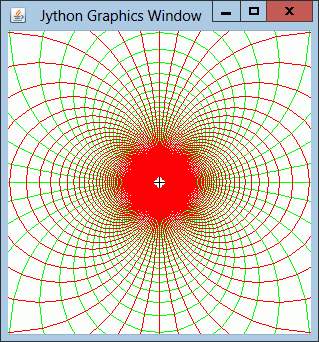Select GPanelEx3.py (Ctrl+C to copy, Ctrl+V to paste)
Execute the program locally using WebStart.

Discussion: To speed up the drawing, we disable the animation by calling enableRepaint(false). Now the graphics is drawn into a background buffer only and rendered to the screen when repaint() is called. (Normally every graphics method calls repaint() automatically, but double-buffering is always enabled to avoid flickering.)

The GPanel supports key and mouse actions. You may poll key actions by calling getKeyCodeWait(). The program halts and waits for any key stroke. When a key is hit, the method returns an integer that represents the key code. (To find a particular code run a test program or use the constants defined in class java.awt.event.KeyEvent.)

```# GPanelEx4.py

from gpanel import *

makeGPanel()
setStatusText("Draw with cursor keys")

x = 0.5
y = 0.5
step = 0.03
move(x, y)
fillCircle(0.01)

while True:
code  = getKeyCodeWait()
if code == 37:  # left cursor key
x = x - step
if code == 38:  # up cursor key
y = y + step
if code == 39:  # right cursor key
x = x + step
if code == 40:  # down cursor key
y = y - step
draw(x, y)
```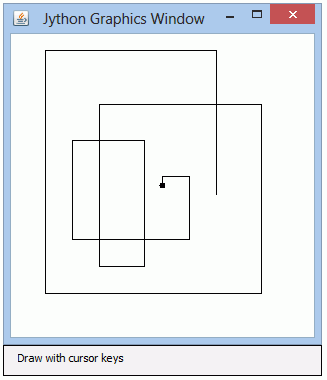Select GPanelEx4.py (Ctrl+C to copy, Ctrl+V to paste)
Execute the program locally using WebStart.

Discussion: The status bar enabled by addStatusBar() is a convenient way to inform the user about the current state of the application.

Mouse actions are handled by callback methods that are fired when a particular mouse event occurs. With Python the callback methods can be registered using named parameters in the makeGPanel()function. The following example implements the well-known problem of "free hand drawing".

```# GPanelEx5.py

from gpanel import *

def onMousePressed(x, y):
global x0, y0
x0 = x
y0 = y

def onMouseDragged(x, y):
global x0, y0
x1 = x
y1 = y
line(x0, y0, x1, y1)
x0 = x1
y0 = y1

makeGPanel(mousePressed = onMousePressed,
mouseDragged = onMouseDragged)
```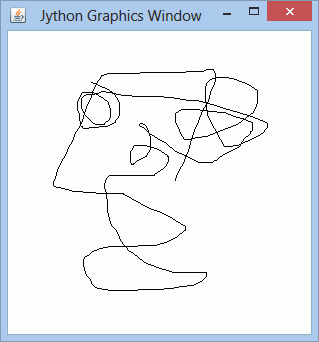Select GPanelEx5.py (Ctrl+C to copy, Ctrl+V to paste)
Execute the program locally using WebStart.

Discussion: In order to register the callback methods, we use the parameters name mousePressed and mouseDragged of the makeGPanel() function. The mouse coordinates x0, y0 must be global variables because they are passed from the onMousePressed() function to the onMouseDragged() function when the mouse button is pressed and between each invocation of the onMouseDragged() function when the mouse is dragged. Enjoy the simplicity of the code.Turtle Graphics GPanel Graphics Lego Robotics Robot Simulation Game Development TCP/IP Imaging Sound Miscellany Download Examples Python Online-Lernplattform (in German, English translation in progress) www.tigerjython.com Python Lernprogramm PHBern (in German) www.jython.ch Python/Jython Home